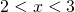# Solution assignment 02 Quadratic inequalities

### Assignment 2

Solve: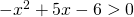### Solution

First we solve the corresponding equation:We can write this equation as: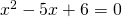which can be solved quite easily by factorizing: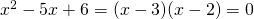and thus the solutions are: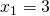or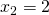The graph of the function in the left-hand side of the inequality is an 'opens down' parabola and thus the following values ofsatisfy the inequality: